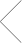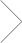# Calculus Tutoring in Carrollton, Texas.

In Home & Online Calculus Tutoring with a Club Z! Tutor.

Get Tutoring Info Now## Our office provides in home and online calculus tutoring services in the following locations including Carrollton, Arbor Creek, Oak Hills Acorn, Indian Creek, Nottingham, Frankford Estates, Park Place, Odca, A. W. Perry, and Addison in Texas.

### Calculus Tutoring: In Home & Online

Club Z! Tutoring of Carrollton, TX provides you with calculus tutors you can count on. Our Club Z! calculus tutors have a minimum of a bachelors degree, and have years of calculus tutoring experience. Many of our calculus tutors even have advanced degrees in mathematics, which will help provide you a full turnkey solution to all of your calculus tutoring needs. Every Club Z! Calculus tutoring session is personalized and unique. In as little as 60 days, Club Z! Students can see an average increase of two letter grades!

Whether it is graphing linear functions or solving differential equations, our calculus tutors are able to help you to excel at every level, including: Pre-Calculus, High School Calculus, AP Calculus, Honors Calculus, and College Level Calculus.

Club Z! Tutoring of Carrollton, TX calculus tutors will help you excel in the subject! We understand that excelling in calculus leads to success in all future math classes, which is why our calculus tutors thoroughly go over each subject to ensure the student has a full understanding of each concept. It doesn’t matter if it is calculus basics or complex problems in college calculus, a Club Z! calculus tutor can help!

In addition to Carrollton, our office offers calculus tutoring services in the following locations: Arbor Creek, Oak Hills Acorn, Indian Creek, Nottingham, Frankford Estates, Park Place, Odca, A. W. Perry, and Addison in Texas.

To find a calculus tutor in your area, please call 469-713-3578 today or fill out our contact form above to speak with an educational specialist.

#### Subjects We TutorElementary Math

Elementary Math Tutoring with a Club Z! Tutor. Club Z! Tutoring of Carrollton, has highly qualified and experienced elementary math tutors who provide one-on-one, focused instruction for all elementary math topics, including addition and subtraction, multiplication, division, fractions, and even Common Core math. Our elementary math tutors will work with your student to not only become proficient, but also a leader in the classroom.Trigonometry

Trigonometry Tutoring with a Club Z! Tutor. Club Z! Tutoring of Carrollton, trigonometry tutors help your student succeed. Our Trigonometry tutors develop personal programs that assess your students trigonometry needs. Our trigonometry Tutors can help your student in all of the following areas of Trigonometry: Oblique triangles, Functions, Right triangle trigonometry and basic identities, Trigonometric identities, Inverse trigonometric, Inverse trigonometric and much more.Calculus

Calculus Tutoring with a Club Z! Tutor. Club Z! Tutoring of Carrollton, provides you with calculus tutors you can count on. Our Club Z! calculus tutors have a minimum of a bachelors degree, and have years of calculus tutoring experience. Whether it is graphing linear functions or solving differential equations, our calculus tutors are able to help you to excel at every level, including: Pre-Calculus, High School Calculus, AP Calculus, Honors Calculus, and College Level Calculus.Geometry

Geometry Tutoring with a Club Z! Tutor. Club Z! Tutoring of Carrollton, geometry tutor develops a unique geometry tutoring program and lesson plan with the student. Our geometry tutors are able to help you excel at every level, including: High School Geometry, Honors Geometry, Middle School Geometry, and College Level Geometry. Our geometry tutors will focus on teaching your student the bestAlgebra

Algebra Tutoring with a Club Z! Tutor. Club Z! Tutoring of Carrollton, algebra tutor develops a unique algebra tutoring program and lesson plan, focused on helping students succeed in algebra. Our algebra tutors prepare their students for algebra tests and quizzes, assist them with algebra homework, help improve classroom performance, and increase their understanding and confidence level in algebra.

Find the right fit or it’s free.

We guarantee you’ll find the right tutor, or we’ll cover the first hour of your lesson.